#### Number of problems found: 514

• A test 2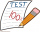A test has 20 questions. The test awards 3 points if the answer is correct and takes away 1 point if the answer is incorrect. A student answered 5 questions incorrectly. How many points did the student earn?​
• One evening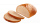One evening, a restaurant served a total of 1/2 of a loaf of wheat bread and 7/8 of a loaf of white bread. How many loaves were served in all?
• MalouMalou spent ₱24.85 for a sandwich and ₱9.20 for juice. How much change did she receive if she gave the vendor ₱100?
• Peter 4Peter has salary 45,55 pesos per hour in a regular day and 2,5 times of regular rate over time. He worked 8 hours per regulars day and 4 hours of overtime. Find total amount of pay receive.
• What is 16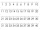What is the sum of three consecutive even integers such that six more than twice the second is 2/3 of the first increased by 3/2 of the third?
• Recurrence relation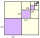Consider a recurrence relation an = 3an-1 - 3an-2 for n = 1,2,3,4,… with initial conditions a1 = 4 and a2 = 2. Calculate a5.
• PLUS MINUS product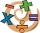A. Deduct 15.728 from the sum of 23.234 and 6.23 B. Add 15.756 to the difference of 26.845 and 4.342 C. Find the product of 0.86 x 0.05 D. Multiply 5.234 by 4.45 E What is the product of 23.97 multiply by 6.36?
• Antonio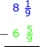Antonio is thinking of an improper fraction and a mixed fraction which have a sum of 5 5/12. The improper fraction is 8/3. What is the mixed number?
• There 19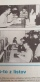There are 19 boys in section A and 23 boys in section B. If 4/7 of them are boy scout, how many boy scouts are there?
• Parentheses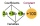Insert parentheses into the expression 2/3 times 5/6 + 3/4 divided by 3/5 so that a. The first operation to be performed is addition and b. The first operation to be performed is division. In both cases evaluate and write results.
• One quarter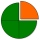Which of the following has a sum of 3/4? A. 1/2+1/4 B. 1/2+1/3 C. 1/4+1/8 D. 1/9+1/12​
• Math: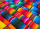Math: solve each problem. 1. for the school's intramurals, a group of students prepared 23 1/2 liters of lemonade to sell. They had 3 5/8 liters leftover. How many liters of lemonade were sold? 2. My mother bought 3 3/4 kg of beef, 23/5 kg of pork, and 5Kate had PHP 368.29. Her mother gave her PHP 253.46 and her sister gave her PHP 57.39. How much money does she has now?
• What is 13What is the number sentence? * 1 point 1/2 + 3/4 + 1/4 = A 1/2 - 3/4 - 1/4 = B 1/2 x 3/4 x 1/4 = C 1/2 ÷ 3/4 ÷ 1/4 = D
• 1700 plans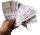1700 plans to budget, 2/5 for rent and 1/6 electricity. What on these two items together?
• Total width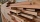Find the total width of 3 boards that 1 ¾ inches wide, 7/8 inch wide, and 1 ½ inches wide.
• The water 3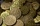The water bill costs Php 875.50 while the phone bill costs Php 1,599.15. How much will be needed to pay the bills?
• Ana bought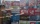Ana bought a bag for Php 350.75 and a blouse worth Php 450.90. How much did she give the cashier if her change was 198.35?
• When Joe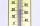When Joe went to work at 6 a. M. , the outside temperature was 3 degrees Celsius. During the day, the Weather worsened and by 3 p. M. the temperature dropped 5 degrees. What was the temperature at 5 p. M. ?
• Spruce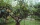A massive storm broke the top of a fifteen-meter spruce so that it remained hanging along the rest of its trunk. The distance of this hanging top from the ground was 4.6 m. At what height was the spruce trunk broken?

Do you have an exciting math question or word problem that you can't solve? Ask a question or post a math problem, and we can try to solve it.

We will send a solution to your e-mail address. Solved examples are also published here. Please enter the e-mail correctly and check whether you don't have a full mailbox.